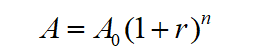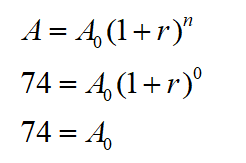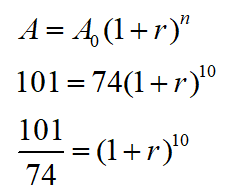# A​ country's populations in 2001and 2011 are given below. Use this information and the exponential model A=P(1+r)n find the growth rate for the country over this period.​population(2001​)= 74 ​millionpopulation(2011)= 101 million The​ country's growth rate was approximately ___​%?(Round to two decimal places as needed)

Question
1 views

A​ country's populations in 2001and 2011 are given below. Use this information and the exponential model A=P(1+r)find the growth rate for the country over this period.

​population(2001​)= 74 ​million
population(2011)= 101 million

The​ country's growth rate was approximately ___​%?
(Round to two decimal places as needed)
check_circle

Step 1

Exponential growth formula isStep 2

Let n represents the number of years since 2001 , when n=0 the the value of A is 72 millionStep 3

Use the value of A_0=74  and find the value of ‘r’. In 2011 the population is 101 million

The value of n=10 in 2011...

### Want to see the full answer?

See Solution

#### Want to see this answer and more?

Solutions are written by subject experts who are available 24/7. Questions are typically answered within 1 hour.*

See Solution
*Response times may vary by subject and question.
Tagged in

### Algebra